# NEET (UG) – 2012 Main Chemistry Previous Year

1. Vapour pressure of chloroform (CHCl3) and dichloromethane (CH2Cl2) at 25°C are 200 mm Hg and 41.5 mm Hg respectively. Vapour pressure of the solution obtained by mixing 25.5 g of CHCl3 and 40 g of CH2Cl2 at the same temperature will be:

(Molecular mass of CHCl3 = 119.5 u and molecular mass of CH2Cl2 = 85 u)

(1) 285.5 mm Hg             (2) 173.9 mm Hg

(3) 615.0 mm Hg             (4) 347.9 mm Hg

2. The Gibbs energy for the decomposition of Al2O3 at 500°C is as follows:

2/3 Al2O3 → 4/3 Al + O2 ;                   ΔrG = + 960kJ mol-1

The potential difference needed for the electrolytic reduction of aluminium oxide (Al2O3) at 500°C at least

(1) 5.0 V                (2) 4.5 V

(3) 3.0 V                (4) 2.5 V

3. Four successive members of the first series of the transition metals are listed below. For which one of them the standard potential (EM2+ /M)value has a positive sign?

(1) Fe (Z = 26)                (2) Co (Z = 27)

(3) Ni (Z = 28)                (4) Cu (Z = 29)

4. Which of the following exhibits only +3 oxidation state?

(1) Pa                     (2) U

(3) Th                    (4) Ac

5. Molar conductivities (˄m0) at infinite dilution of NaCl, HCl and CH3COONa are 126.4, 425.9 and 91.0 S cm2 mol–1 respectively. ˄m0 for CH3COOH will be:

(1) 390.5 S cm2 mol–1                (2) 425.5 S cm2 mol–1

(3) 180.5 S cm2 mol–1                (4) 290.8 S cm2 mol–1

6. In which of the following arrangements the given sequence is not strictly according to the property indicated against it?

(1) CO2 < SiO2 < SnO2 < PbO2 : increasing oxidising power

(2) HF < HCl < HBr < HI : increasing acidic strength

(3) H2O < H2S < H2Se < H2Te : increasing pKa values

(4) NH3 < PH3 < AsH3 < SbH3 : increasing acidic character

8. During change of O2 to O2 ion, the electron adds on which one of the following orbitals?

(1) σ orbital (2) π* orbital (3) π orbital (4) σ* orbital

9. Standard reduction potentials of the half reactions are given below:

F2(g) + 2e → 2F  (aq);            E0 = +2.85V

Cl2(g) + 2e → 2Cl  (aq);         E0 = +1.36 V

Br2(g) + 2e → 2Br  (aq);         E0 = +1.06 V

I2(g) + 2e → 2I (aq) ;              E0 = +0.53 V

The strongest oxidising and reducing agents respectively are:

(1) Cl2 and I2                   (2) F2 and I

(3) Br2 and Cl                 (4) Cl2 and Br

10. The catalytic activity of transition metals and their compounds is ascribed mainly to

(1) their chemical reactivity

(2) their magnetic behaviour

(3) their unfilled d-orbitals

(4) their ability to adopt variable oxidation states

11. Equal volumes of two monoatomic gases, A and B, at same temperature and pressure are mixed. The ratio of specific heats (Cp/Cv) of the mixture will be

(1) 1.67                 (2) 0.83

(3) 1.50                 (4) 3.3

12. Given that the equilibrium constant for the reaction

2SO2 (g) + O2 (g) ⇌ 2SO3(g)

has a value of 278 at a particular temperature. What is the value of the equilibrium constant for the following reaction at the same temperature.

2SO3 (g) ⇌ 1/2 O2 (g) 2SO2(g)

(1) 1.3 × 10–5                   (2) 1.8 × 10–3

(3) 3.6 × 10–3                   (4) 6.0 × 10–2

13. Which one of the following sets forms the Bidegradable polymer?

(a)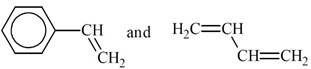(b)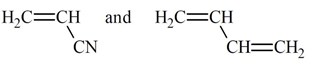(c)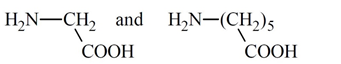(d)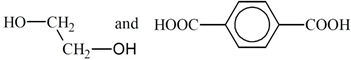14.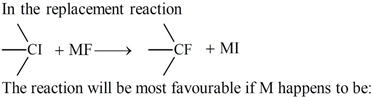(1) Li                (2) Na          (3) K            (4) Rb

15. Activation energy (Ea) and rate constants (k1 and k2) of a chemical reaction of two different temperatures (T1 and T2) are related by:

(a)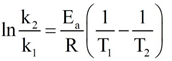(b)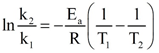(c)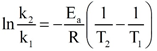(d)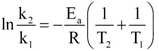16. Which one of the following does not correctly represent the correct order of the property indicated against it?

(1) Ti < V < Mn < Cr : increasing 2nd ionization enthalpy

(2) Ti < V < Cr < Mn : increasing number of oxidation states

(3) Ti3+ < V3+ < Cr3+ < Mn3+ : increasing magnetic moment

(4) Ti < V < Cr < Mn : increasing melting points

17. Red precipitate is obtained when ethanol solution of dimethylglyoxime is added to ammoniacal Ni(II). Which of the following statements is not true?

(1) Dimethylglyoxime functions as bidentate ligand

(2) Red complex has a square planar geometry

(3) Complex has symmetrical H-bonding

(4) Red complex has a tetrahedral geometry

18. An organic compound (C3H9N) (A), when treated with nitrous acid,
gave an alcohol and N2 gas was evolved. (A) on warming with CHCl3
and caustic potash gave (C) which on reduction gave isopropylmethylamine.
Predict the structure (A).

(a)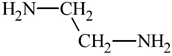(b)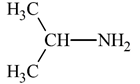(c)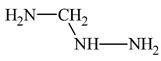(d)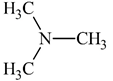19. Structure of a mixed oxide is cubic close-packed (c.c.p). The cubic unit cell of mixed oxide is composed of oxide ions. One fourth of the tetrahedral voids are occupied by divalent metal A and the octahedral voids are occupied by a monovalent metal B. The formula of the oxide is:

(1) AB2O2                  (2) ABO2

(3) A2BO2                  (4) A2B3O4

20. The orbital angular momentum of a p-electron is given as:

(a)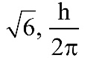(b)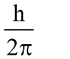(c)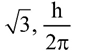(d)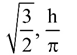21. Four diatomic species are listed below. Identify the correct order in which the bond order is increasing in them:

(1) He2+ < O2 < NO < C22-

(2) NO < O2 < C22- < He2+

(3) O2 < NO < C22- < He2+

(4) C22- < He2+ < O2 < NO

22. Which of the following compounds can be used as antifreeze in automobile radiators?

(1) Ethyl alcohol              (2) Methyl alcohol

(3) Glycol                        (4) Nitrophenol

23.